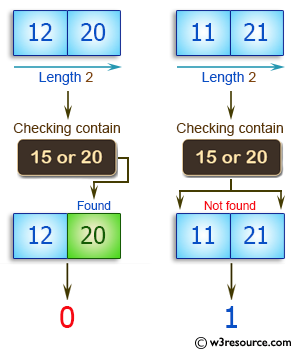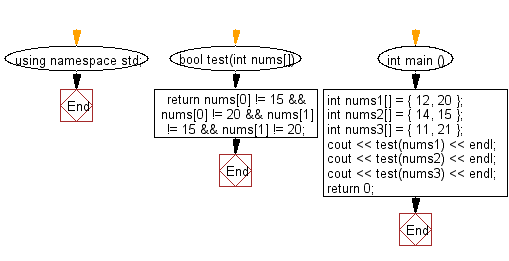﻿ C++ : Check if an array of size 2, does not contain 15 or 20# C++ Exercises: Check if a given array of integers and length 2, does not contain 15 or 20

## C++ Basic Algorithm: Exercise-90 with Solution

Write a C++ program to check if a given array of integers and length 2, does not contain 15 or 20.

Sample Solution:

C++ Code :

``````#include <iostream>

using namespace std;

bool test(int nums[]) {
return nums != 15 && nums != 20 && nums != 15 && nums != 20;
}

int main () {
int nums1[] = { 12, 20 };
int nums2[] = { 14, 15 };
int nums3[] = { 11, 21 };
cout << test(nums1) << endl;
cout << test(nums2) << endl;
cout << test(nums3) << endl;
return 0;
}
``````

Sample Output:

```0
0
1
```

Pictorial Presentation:Flowchart:C++ Code Editor:

Contribute your code and comments through Disqus.

What is the difficulty level of this exercise?

﻿